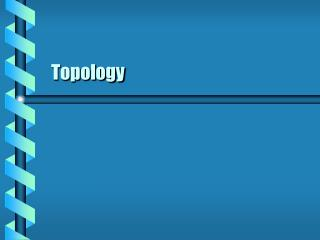DownloadDownload PresentationTopology

# Topology

Download Presentation## Topology

- - - - - - - - - - - - - - - - - - - - - - - - - - - E N D - - - - - - - - - - - - - - - - - - - - - - - - - - -
##### Presentation Transcript

1. Topology

2. Uniformly Continuous • The field of topology is about the geometrical study of continuity. • A map f from a metric space (X, d) to a metric space (Y, D) is continuous if: • Given e > 0, d > 0, • If d(x1, x2) < d, then d(f(x1), f(x2)) < e.

3. Sequences • Map from positive integers to a set; s: Z+→X • nth point is xn s(n) • Sequence {xn} converges to y • given e > 0 there exists m • d(xn, y) < e for nm • Limit point p in YX • There exists a sequence of points in Y converging to p X yn y1 p Y

4. A set YX is closed if it contains all its limit points. The closure of Y, cl(Y) is the set of all limit points in Y. Ball of radius r centered at x B(x, r) = {y: yX, d(x, y) < r} A set UX is open For each xUr The ball B(x, r)  U Radius r may depend on x Open and Closed Closed set includes its boundary Open set missing its boundary

5. The interior of SY Int(S) = Y – cl(Y - S) The neighborhood of a point xX NX x int(N) Neighborhood Y Int(S) N x X

6. A function f is continuous if X and Y are metric spaces The function f: XY The function f-1: YX  open VY, f-1(V) is open A homeomorphism f If f is continuous and f is invertible and f-1 is continuous Homeomorphism X f f-1 V Y

7. Scalar field maps from a space to the real numbers. F = f(x1, x2, x3) A constraint can reduce the variables to a surface. F(x1, x2, x3) = 0 F = f(u1, u2) Smooth fields are measured by their differentiability. Cn-smooth is n times differentiable Smoothness F is not C2smooth is C2 smooth

8. A function f is class Cn XEa and YEb The function f: XY Open sets UX, VY The function F: UV Partial derivatives of F are continuous to order n F and f agree on X A function fis smooth Class Cn for all n fis a Cn-diffeomorphism and f is invertible and f-1 is class Cn Diffeomorphism U F X f Y V next